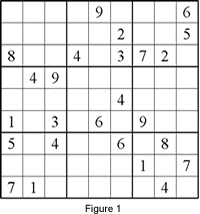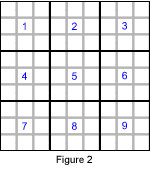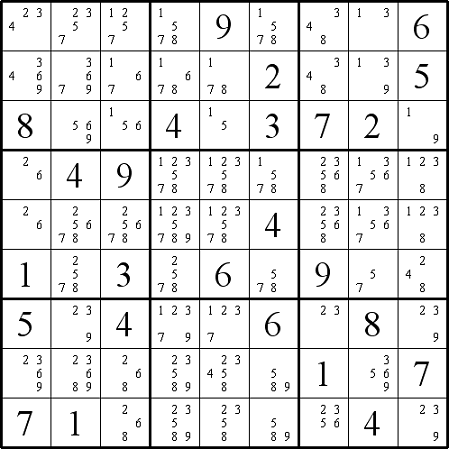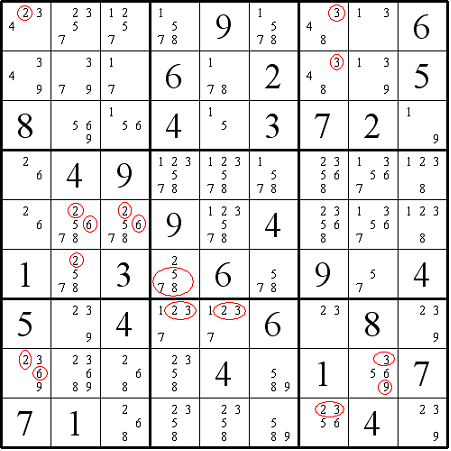# The Naked Pair in Sudoku

##### Age 7 to 16

Published 2006 Revised 2011

This article describes a particular strategy useful in solving Sudoku puzzles known as "the naked pair". The discussions will be based on the Sudoku shown in Figure 1, and which can also be downloaded here .

 The game of Sudoku consists of a grid of nine rows and nine columns subdivided into nine 3x3 subgrids. It has two basic rules: Each column, each row, and each box (3x3 subgrid) must have the numbers 1 to 9. No column, row or box can have two squares with the same number.To help locate boxes (squares of 9 cells that contain each of the digits 1-9), I have numbered them from 1-9 as shown in Figure 2. Individual cells are located using the notation (row, column), that is row number followed by the column number. In Figure1 (1,5) contains the number 9.

In solving any puzzle, the first thing we can do is find all the available single candidates in the rows, columns and boxes. That is, individual cells that are the only place where particular numbers can be put.

To explain this a little more, let's look at Figure 3 where we have listed all the possible numbers that can fit into each cell (taking into account what already appears in the rows, columns and boxes). We can now find four single candidates by the method of "force" .

These single candidates are:
6 in cell (2,4) because there is nowhere else in this block (and column) that the 6 can go,
9 in cell (5,4) because this is the only cell in this block (and row) where the 9 can be placed,
4 in cell (6,9), the only cell in this block, row and column that 4 can be placed and, similarly,
4 in cell (8,5).#### Figure 3

We can now remove:
all the other 6's in row 2 and all the other 9's in column 4 (see Figure 4).

After finding all the available single candidates, we can start tackling all the naked pairs.

Looking at Figure 4, the cells (4,1) and (5,1) in column 1 have the same two candidates 2 and 6, forming a naked pair. This means that the two cells can be the only place for 2 and 6 in the same column and the same box. As a result, the options 2 and 6 can be removed from the candidates of the other cells in the same column and the same box. So 2 can be removed from cell (1,1), and 2 and 6 can be removed from cell (8, 1). As cells (4,1) and (5,1) belong to box 4, the candidates 2 and 6 can also be removed from cells (5,2) and (5,3). Likewise, the candidate 2 can be removed from cell (6,2).Figure 4

The naked pair with the candidate numbers of 2 and 6 was easy to spot. However, a naked pair can often be found hiding as a "hidden pair" among other redundant candidate numbers. There is one such example in Figure 4. We can find the numbers 1 and 7 in box 7 and box 9 and in both the last two rows. This means that no other empty cell in the last 3 rows, except the cells (7,4) and (7,5) can contain the naked pair of 1 and 7 as a solution.

When we are in a hurry, especially during a competition, we tend to pencil in redundant options. For example in Figure 3, the two cells (7,4) and (7,5) contain 2, 3 and 9 as well as 1 and 7 which, as shown, are the only two candidate numbers. This results in a change from 1 and 7 being a naked pair to a hidden pair. Hence it is a good practice to make a note, for example by circling the redundant clue numbers (Figure 4). The same rule can be applied to instances of more than two cells, e.g. "naked triplets" and "naked quads".

In row 6, the only position possible for a 2 is (6, 4). See the paragraph above Figure 4 if you cannot see why the 2 in (6,2) cannot be used. This means that the three cells (6,2), (6,6) and (6,8) in row 6 form a naked triplet with the candidate numbers 5, 7 and 8.

The three cells (7,7), (7,9) and (9,9) in box 9 form another naked triplet with the candidate numbers 2, 3 and 9. Hence the redundant options 2 and 3 can be removed from cell (9,7). Similarly, the redundant options 3 and 9 can be removed from cell (8,8). As a result, the cells (9,7) and (8,8) form a new naked pair with the candidate numbers 5 and 6.

Finally, the three cells (1,8), (2,8) and (3,9) in box 3 form a naked triplet with the candidate numbers 1, 3 and 9. This means the redundant option 3 can be removed from cells (1,7) and (2,7) forming a naked pair with the candidate numbers 4 and 8.

A puzzle consisting of only single candidates and naked pairs should be classified under the easy category. After all the redundant candidates in the empty cells are removed by the technique of "naked pair" new single candidates begin to appear in the puzzle.

The rest of the puzzle can be easily solved by basic techniques. I leave the rest of the solution to the readers.

A second sudoku article can be found here.